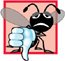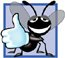# Relationship Between Pointers and Arrays

Arrays and pointers are intimately related in C++ and may be used almost interchangeably. An array name can be thought of as a constant pointer. Pointers can be used to do any operation involving array subscripting.

Assume the following declarations:

```int b[ 5 ]; // create 5-element int array b
int *bPtr; // create int pointer bPtr
```

Because the array name (without a subscript) is a (constant) pointer to the first element of the array, we can set bPtr to the address of the first element in array b with the statement

```bPtr = b; // assign address of array b to bPtr
```

This is equivalent to taking the address of the first element of the array as follows:

```bPtr = &b[ 0 ]; // also assigns address of array b to bPtr
```

Array element b[ 3 ] can alternatively be referenced with the pointer expression

```*( bPtr + 3 )
```

The 3 in the preceding expression is the offset to the pointer. When the pointer points to the beginning of an array, the offset indicates which element of the array should be referenced, and the offset value is identical to the array subscript. The preceding notation is referred to as pointer/offset notation. The parentheses are necessary, because the precedence of * is higher than the precedence of +. Without the parentheses, the above expression would add 3 to the value of *bPtr (i.e., 3 would be added to b[ 0 ], assuming that bPtr points to the beginning of the array). Just as the array element can be referenced with a pointer expression, the address

```&b[ 3 ]
```

can be written with the pointer expression

```bPtr + 3
```

The array name can be treated as a pointer and used in pointer arithmetic. For example, the expression

```*( b + 3 )
```

also refers to the array element b[ 3 ]. In general, all subscripted array expressions can be written with a pointer and an offset. In this case, pointer/offset notation was used with the name of the array as a pointer. Note that the preceding expression does not modify the array name in any way; b still points to the first element in the array.

Pointers can be subscripted exactly as arrays can. For example, the expression

```bPtr[ 1 ]
```

refers to the array element b[ 1 ]; this expression uses pointer/subscript notation.

Remember that an array name is a constant pointer; it always points to the beginning of the array. Thus, the expression

```b += 3
```

causes a compilation error, because it attempts to modify the value of the array name (a constant) with pointer arithmetic.

Common Programming Error 8.14Although array names are pointers to the beginning of the array and pointers can be modified in arithmetic expressions, array names cannot be modified in arithmetic expressions, because array names are constant pointers.

Good Programming Practice 8.2For clarity, use array notation instead of pointer notation when manipulating arrays.

Figure 8.20 uses the four notations discussed in this section for referring to array elementsarray subscript notation, pointer/offset notation with the array name as a pointer, pointer subscript notation and pointer/offset notation with a pointerto accomplish the same task, namely printing the four elements of the integer array b.

Figure 8.20. Referencing array elements with the array name and with pointers.

(This item is displayed on pages 428 - 429 in the print version)

``` 1 // Fig. 8.20: fig08_20.cpp
2 // Using subscripting and pointer notations with arrays.
3 #include
4 using std::cout;
5 using std::endl;
6
7 int main()
8 {
9 int b[] = { 10, 20, 30, 40 }; // create 4-element array b
10 int *bPtr = b; // set bPtr to point to array b
11
12 // output array b using array subscript notation
13 cout << "Array b printed with:

Array subscript notation
";
14
15 for ( int i = 0; i < 4; i++ )
16 cout << "b[" << i << "] = " << b[ i ] << '
';
17
18 // output array b using the array name and pointer/offset notation
19 cout << "
Pointer/offset notation where "
20 << "the pointer is the array name
";
21
22 for ( int offset1 = 0; offset1 < 4; offset1++ )
23 cout << "*(b + " << offset1 << ") = " << *( b + offset1 ) << '
';
24
25 // output array b using bPtr and array subscript notation
26 cout << "
Pointer subscript notation
";
27
28 for ( int j = 0; j < 4; j++ )
29 cout << "bPtr[" << j << "] = " << bPtr[ j ] << '
';
30
31 cout << "
Pointer/offset notation
";
32
33 // output array b using bPtr and pointer/offset notation
34 for ( int offset2 = 0; offset2 < 4; offset2++ )
35 cout << "*(bPtr + " << offset2 << ") = "
36 << *( bPtr + offset2 ) << '
';
37
38 return 0; // indicates successful termination
39 } // end main
```

 ``` Array b printed with: Array subscript notation b = 10 b = 20 b = 30 b = 40 Pointer/offset notation where the pointer is the array name *(b + 0) = 10 *(b + 1) = 20 *(b + 2) = 30 *(b + 3) = 40 Pointer subscript notation bPtr = 10 bPtr = 20 bPtr = 30 bPtr = 40 Pointer/offset notation *(bPtr + 0) = 10 *(bPtr + 1) = 20 *(bPtr + 2) = 30 *(bPtr + 3) = 40 ```

To further illustrate the interchangeability of arrays and pointers, let us look at the two string-copying functionscopy1 and copy2in the program of Fig. 8.21. Both functions copy a string into a character array. After a comparison of the function prototypes for copy1 and copy2, the functions appear identical (because of the interchangeability of arrays and pointers). These functions accomplish the same task, but they are implemented differently.

Figure 8.21. String copying using array notation and pointer notation.

``` 1 // Fig. 8.21: fig08_21.cpp
2 // Copying a string using array notation and pointer notation.
3 #include
4 using std::cout;
5 using std::endl;
6
7 void copy1( char *, const char * ); // prototype
8 void copy2( char *, const char * ); // prototype
9
10 int main()
11 {
12 char string1[ 10 ];
13 char *string2 = "Hello";
14 char string3[ 10 ];
15 char string4[] = "Good Bye";
16
17 copy1( string1, string2 ); // copy string2 into string1
18 cout << "string1 = " << string1 << endl;
19
20 copy2( string3, string4 ); // copy string4 into string3
21 cout << "string3 = " << string3 << endl;
22 return 0; // indicates successful termination
23 } // end main
24
25 // copy s2 to s1 using array notation
26 void copy1( char * s1, const char * s2 )
27 {
28 // copying occurs in the for header
29 for ( int i = 0; ( s1[ i ] = s2[ i ] ) != ''; i++ )
30  ; // do nothing in body
31 } // end function copy1
32
33 // copy s2 to s1 using pointer notation
34 void copy2( char *s1, const char *s2 )
35 {
36 // copying occurs in the for header
37 for ( ; ( *s1 = *s2 ) != ''; s1++, s2++ )
38  ; // do nothing in body
39 } // end function copy2
```

 ``` string1 = Hello string3 = Good Bye ```

Function copy1 (lines 2631) uses array subscript notation to copy the string in s2 to the character array s1. The function declares an integer counter variable i to use as the array subscript. The for statement header (line 29) performs the entire copy operationits body is the empty statement. The header specifies that i is initialized to zero and incremented by one on each iteration of the loop. The condition in the for, ( s1[ i ] = s2[ i ] ) != '', performs the copy operation character by character from s2 to s1. When the null character is encountered in s2, it is assigned to s1, and the loop terminates, because the null character is equal to ''. Remember that the value of an assignment statement is the value assigned to its left operand.

Function copy2 (lines 3439) uses pointers and pointer arithmetic to copy the string in s2 to the character array s1. Again, the for statement header (line 37) performs the entire copy operation. The header does not include any variable initialization. As in function copy1, the condition ( *s1 = *s2 ) != '' performs the copy operation. Pointer s2 is dereferenced, and the resulting character is assigned to the dereferenced pointer s1. After the assignment in the condition, the loop increments both pointers, so they point to the next element of array s1 and the next character of string s2, respectively. When the loop encounters the null character in s2, the null character is assigned to the dereferenced pointer s1 and the loop terminates. Note that the "increment portion" of this for statement has two increment expressions separated by a comma operator.

The first argument to both copy1 and copy2 must be an array large enough to hold the string in the second argument. Otherwise, an error may occur when an attempt is made to write into a memory location beyond the bounds of the array (recall that when using pointer-based arrays, there is no "built-in" bounds checking). Also, note that the second parameter of each function is declared as const char * (a pointer to a character constanti.e., a constant string). In both functions, the second argument is copied into the first argumentcharacters are copied from the second argument one at a time, but the characters are never modified. Therefore, the second parameter is declared to point to a constant value to enforce the principle of least privilegeneither function needs to modify the second argument, so neither function is allowed to modify the second argument.C++ How to Program (5th Edition)
ISBN: 0131857576
EAN: 2147483647
Year: 2004
Pages: 627